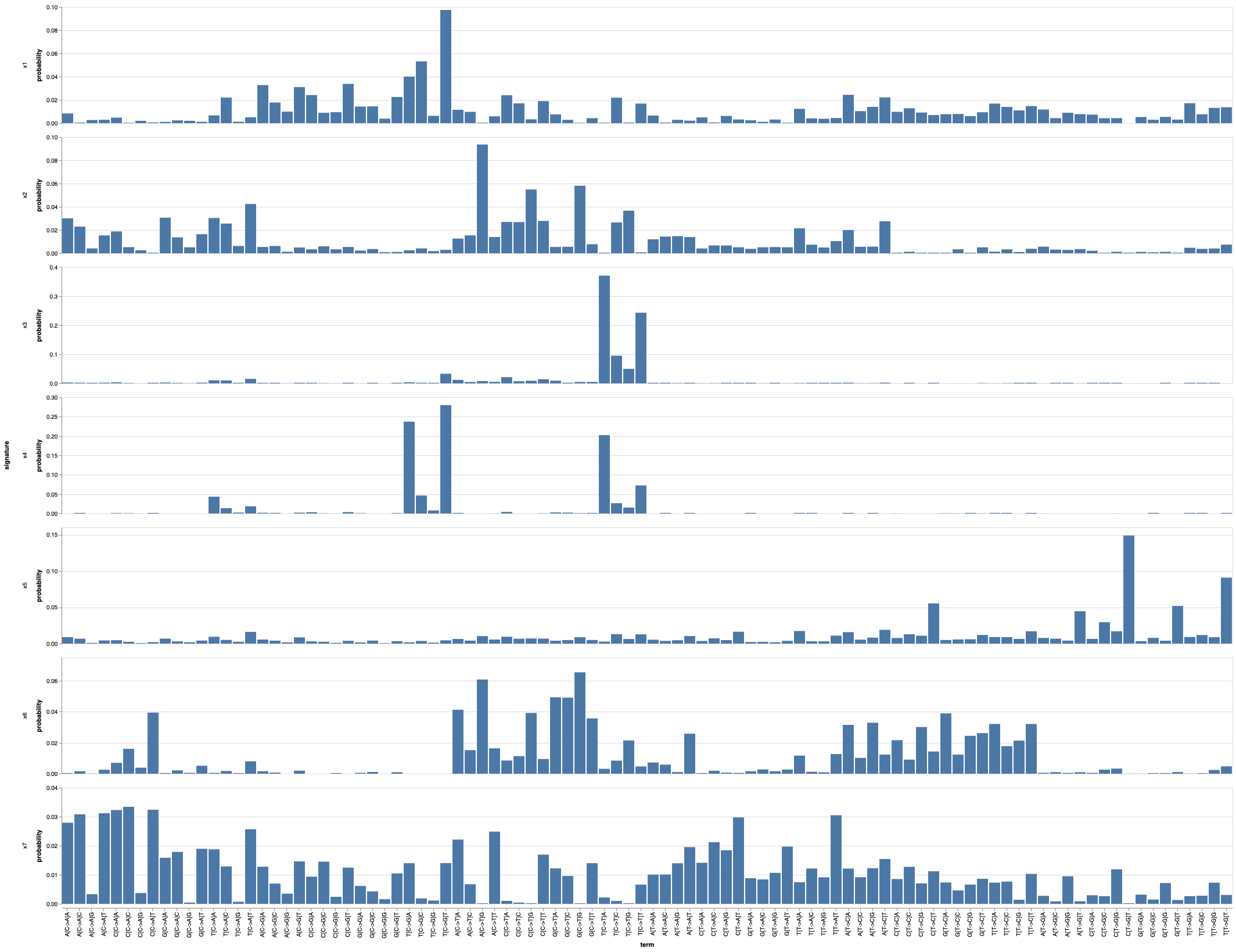Fork me on GitHub!

MultiModalMuSig

A Julia package implementing several topic models used for mutation signature estimation.

The Multi-modal correlated topic model (MMCTM) takes an array of arrays of matrices, where the first column of each matrix is a mutation type index, and the second column is the mutation count for a particular sample.

The following example shows how to perform inference using the MMCTM on SNV and SV counts:

using MultiModalMuSig
using CSV
using DataFrames
using VegaLite
using Random

Random.seed!(42)

snv_counts = CSV.read("data/brca-eu_snv_counts.tsv", delim='\t')
sv_counts = CSV.read("data/brca-eu_sv_counts.tsv", delim='\t')

X = format_counts_mmctm(snv_counts, sv_counts)
model = MMCTM([7, 7], [0.1, 0.1], X)
fit!(model, tol=1e-5)

snv_signatures = DataFrame(hcat(model.ϕ...))
sv_signatures = DataFrame(hcat(model.ϕ...))

snv_signatures[:term] = snv_counts[:term]
snv_signatures = melt(
snv_signatures, :term, variable_name=:signature, value_name=:probability
)
snv_signatures |> @vlplot(
:bar, x={:term, sort=:null}, y=:probability, row=:signature,
resolve={scale={y=:independent}}
)This code runs the MMCTM for 7 SNV and 7 SV signatures, with signature hyperparameters set to 0.1. Since these types of models can get stuck in poor local optima, it’s a good idea to fit many models and pick the best one.

Sample-signature probabilities can be extracted like so:

# sample 3, SNV signature probabilities (modality 1)
model.props
# SV signature probabilities (modality 2)
model.props

# SNV probabilities for all samples
snv_props = hcat(
[model.props[i] for i in 1:length(model.props)]...
)

The MMCTM can be run on multiple modalities, e.g.

X = format_counts_mmctm(snv_counts, sv_counts, indel_counts)
model = MMCTM([7, 7, 5], [0.1, 0.1, 0.1], X)

The DataFrame inputs to format_counts_mmctm have an optional term column, and further columns for each sample.

To run the CTM instead, just run the MMCTM with a single modality:

X = format_counts_ctm(snv_counts)
model = MMCTM(, [0.1], X)
fit!(model, tol=1e-5)

The LDA implementation can be run like so:

X = format_counts_lda(snv_counts)
model = LDA(7, 0.1, 0.1, X)
fit!(model, tol=1e-5)

In the above code, both the sample-signature and signature-term hyperparameters have been set to 0.1, respectively. After fitting LDA, signatures can be found in model.β, and signature probabilities can be found in model.θ.